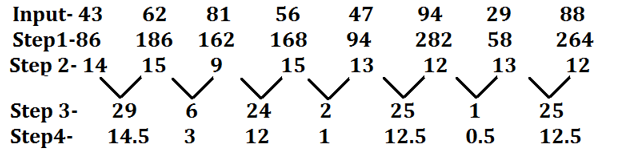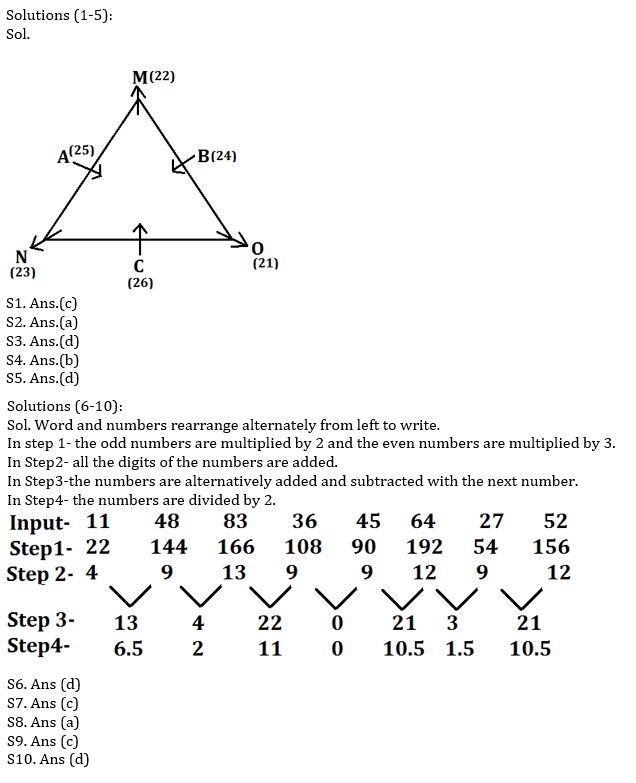Latest Banking jobs   »   Reasoning Ability Quiz For RRB PO...

# Reasoning Ability Quiz For RRB PO Mains 2021- 28th August

Directions (1-5): Study the information carefully and answer the questions given below.
Six persons are sitting around a triangular table, in such a way three persons are sitting three corners facing outside while three are sitting at middle of the side facing inside. Each of them celebrates their birthday on different date 21 to 26 of the same month of the sane year. A sits second to the left of C who is younger than A. Only two persons are sitting between N and B. O is older than B. N is 2days younger than O. M sits second to the right of N. B is not an immediate neighbour of A. Youngest person sits at the middle of the side. B is younger than N but not youngest person. A celebrate his birthday on an odd number date but not on prime number date. Age difference between M and C is 4 days.

Q1. Who among the following celebrate his birthday on 22nd?
(a) C
(b) O
(c) M
(d) N
(e) None of these

Q2. Who among the following person sits second to the right of C?
(a) B
(b) O
(c) M
(d) A
(e) None of these

Q3. How many persons sit between N and M, when counted left of M?
(a) More than three
(b) Two
(c) Three
(d) One
(e) None

Q4. Who among the following is oldest person?
(a) B
(b) O
(c) M
(d) A
(e) None of these

Q5. Who among the following sits third to the right of the second youngest person?
(a) N
(b) C
(c) B
(d) O
(e) None of these

Directions (6-10): Read the following information carefully and answer the questions given below.
A number arrangement machine when given an input line of numbers, rearranges them following a particular rule in each step. The following is an illustration of input and rearrangement.Step IV is the last step of the above arrangement as the intended arrangement is obtained.
As per the rules followed in the given steps find out the appropriate steps for the given input:

Input: 11 48 83 36 45 64 27 52

Q6. Which of the following will be Step III for the above input?
(a) 13 4 22 0 21 3 22
(b) 13 4 22 0 22 3 21
(c) 13 4 22 1 21 3 21
(d) 13 4 22 0 21 3 21
(e) None of these

Q7. Which of the following is 2nd from the left end in last step?
(a) 1.5
(b) 0
(c) 2
(d) 6.5
(e) None of these

Q8.What is the sum of the numbers which is 3rd from the right in step 1 and 2nd from the left in last step?
(a) 194
(b) 34
(c) 267
(d) 65
(e) None of these

Q9 What is the difference between the numbers which is 3rd from the right in step 3 and 2nd from the left in step 2?
(a) 9
(b) 4
(c) 12
(d) 6
(e) None of these

Q10.How many numbers are exactly divisible by 3 in step 1?
(a) Two
(b) Four
(c) Five
(d) Six
(e) None of these

Solutions